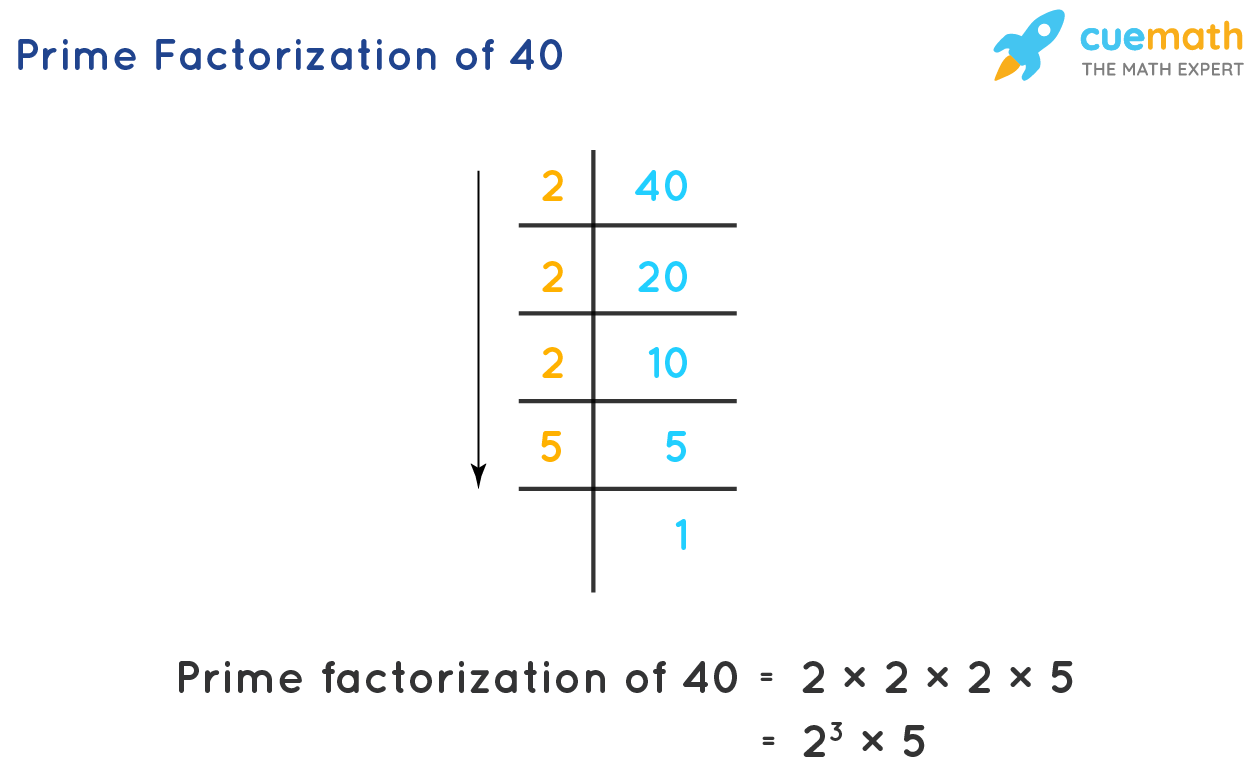Fri. Oct 22nd, 2021

# What is so Trendy About Prime Factorization?Many of the students might be having great interest in a subject like Mathematics. It is a subject that will help them to know more about how different things are calculated and how each concept of mathematics can be applied in real life to get the solutions. Once the students come to the high school, they become familiar with the interesting topic like Prime factorization.

Now the first question arises what is prime factorization and what does it do? It is a way of expressing the number as a product that is known as the prime factor. The prime numbers are the numbers that have only two factors i.e 1 and the number itself. For example, we take the number 7. It has only two factors i.e. 1 and 7, so it is a prime number. The smallest prime number is the 2.

For understanding the prices of prima factorizations, we take the example of number 30. We all know that 5*6 comes out to be 30. Here 6 is not a prime number, so 6 is the product of two numbers i.e. 2 and 3. Therefore the prime factorizations will comes out to be 2*3*5= 30.

So basically the process of writing the numbers as the product of the prime numbers is known as the prime factorization. Many prime numbers start from 2 followed by 3, 5, 7, 11, 13, 17, 19, 23, 31, and the list continues. To calculate the prime factorization of the given number, two commonly used methods make it easy for the student to calculate it i.e. Prime factorization using factor tree methods and Division method of Prime Factorization.

• Prime Factorization using Factor Tree method: In this, the student can follow certain steps given below:
1. First of all the student needs to understand the given numbers as the root of the tree, that comes at the top of the tree factor.
2. Then the corresponding pair of factors are written in the form of the branches of the tree.
3. All the composite numbers are factorized to such an extent that all the prime factors list comes out on the branches of the tree.
4. In the end, multiplication is done of all the prime factors to get the final answer.

For example, the student is given the number 600, to find the prime factorization.  The two highest factors will be 20 and 30, so both these are composite numbers, so the student needs to factorize it further. 20 will give again two factors 4 and 5. On the other hand, 30 will give two factors 6 and 5. Now there is only one composite number left in 20 factors i.e. 4 that gives two factors 2 and 2 whereas 6 from 30 gives two factors 2 and 3. Now the student is left with all the prime factors. The answer will be 2*2*2*3*5*5 = 600.

• Division method of Prime factorization: In this method of factorizations, the numbers are divided with prime numbers, so simplify the given number. In this the student can follow certain steps given below:
1. First of all the student needs to divide the number with the smallest prime number correctly to get the simplified number.
2. Again the simplified number is divided into the smallest prime factor, this process is repeated until the quotient becomes 1.
3. Finally in the end the person needs to multiples all the prime factors that are the divisors of in this division to get the final result.

There are different range of properties of Prime factorization given below:

• Cryptography and Prime factorization: It is a method of protecting the information and communicating them in the code language. In this, it plays a very important role to provide great help in the usage of different computers.
• HCF and LCM usingprime factorization: To evaluate HCF and LCM of the two numbers, prime factorization plays a very important role.

So, if the student has the in-depth knowledge about the prime factorization and the multiples involved in find that, it can become an easy task for them. If it seems tough task, they can always take an expert help from Cuemath, an excellent online coaching platform.